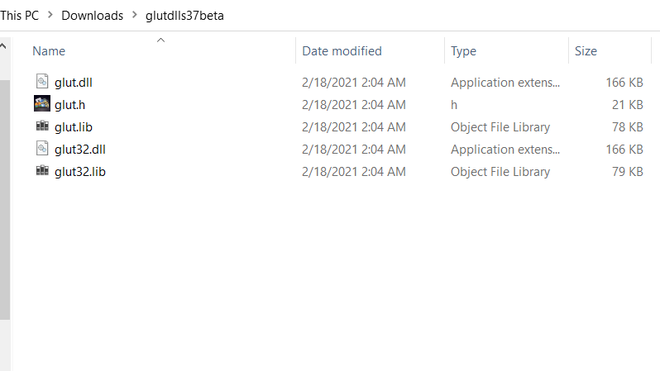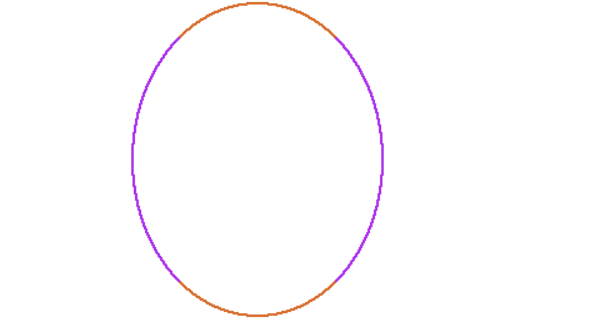Related Articles
How to setup OpenGL with Visual Studio 2019 on Windows 10?
• Difficulty Level : Hard
• Last Updated : 01 Mar, 2021

OpenGL is a 2D and 3D graphics API that provides a cross-platform application programming interface for a wide variety of computer platforms. It is operating system independent and has a transparent network. Application developers use it to develop high-performing and visually compelling graphics software applications.

### Installation:

Now let’s jump into the setup of OpenGL. To do so follow the below steps:

Step 1: First we have to download Visual Studio 2019 for windows10.

Step 2: Now we have installed Visual Studio2019 from its downloaded the .exe file with Windows installer.Select Visual Stdio community 2019 and click Modify, now select  the requited components as shown in below image and click install while Downloading :It will take some time after it will be complete  restart the computer after the installation take place.

Step 3:

• Now download GLUT header file, the .LIB, and .DLL files all pre-compiled for Intel platforms, you can simply download the glutdlls37beta.zip file from the website.
• File inside folder look like this:Step 4: After this, we have to copy the file as instructed below:

• First, paste glut.h file in:
`C:\Program Files (x86)\Microsoft Visual Studio\2019\Community\VC\Auxiliary\VS\include\gl`
• Then paste glut.lib in:
` C:\Program Files (x86)\Microsoft Visual Studio\2019\Community\VC\Auxiliary\VS\lib\64`
• Then paste the glut32.lib in:
`C:\Program Files (x86)\Microsoft Visual Studio\2019\Community\VC\Auxiliary\VS\lib\86`
• Then paste glut.dll and glut32.dll in:
` C:\Windows\SysWOW64`
• Finally  copy glut32.dll to:
`C:\Windows\System32 `

Take a look at the below video for installation:

Here is one sample code for running to check whether it is working or not

## C++

 `#include ``#include``using` `namespace` `std;``int` `rx = 100, ry = 125;``int` `xCenter = 250, yCenter = 250;` `void` `myinit(``void``)``{``    ``glClearColor(1.0, 1.0, 1.0, 0.0);``    ``glMatrixMode(GL_PROJECTION);``    ``glLoadIdentity();``    ``gluOrtho2D(0.0, 640.0, 0.0, 480.0);``}` `void` `setPixel(GLint x, GLint y)``{``    ``glBegin(GL_POINTS);``    ``glVertex2i(x, y);``    ``glEnd();``}``void` `ellipseMidPoint()``{``    ``float` `x = 0;``    ``float` `y = ry;``    ``float` `p1 = ry * ry - (rx * rx) * ry + (rx * rx) * (0.25);``    ``float` `dx = 2 * (ry * ry) * x;``    ``float` `dy = 2 * (rx * rx) * y;``    ``glColor3ub(``rand``() % 255, ``rand``() % 255, ``rand``() % 255);``    ``while` `(dx < dy)``    ``{``        ``setPixel(xCenter + x, yCenter + y);``        ``setPixel(xCenter - x, yCenter + y);``        ``setPixel(xCenter + x, yCenter - y);``        ``setPixel(xCenter - x, yCenter - y);``        ``if` `(p1 < 0)``        ``{``            ``x = x + 1;``            ``dx = 2 * (ry * ry) * x;``            ``p1 = p1 + dx + (ry * ry);``        ``}``        ``else``        ``{``            ``x = x + 1;``            ``y = y - 1;``            ``dx = 2 * (ry * ry) * x;``            ``dy = 2 * (rx * rx) * y;``            ``p1 = p1 + dx - dy + (ry * ry);``        ``}``    ``}``    ``glFlush();` `    ``float` `p2 = (ry * ry) * (x + 0.5) * (x + 0.5) + (rx * rx) * (y``        ``- 1) * (y - 1) - (rx * rx) * (ry * ry);``    ``glColor3ub(``rand``() % 255, ``rand``() % 255, ``rand``() % 255);``    ``while` `(y > 0)``    ``{``        ``setPixel(xCenter + x, yCenter + y);``        ``setPixel(xCenter - x, yCenter + y);``        ``setPixel(xCenter + x, yCenter - y);``        ``setPixel(xCenter - x, yCenter - y);``        ``if` `(p2 > 0)``        ``{``            ``x = x;``            ``y = y - 1;``            ``dy = 2 * (rx * rx) * y;``            ``p2 = p2 - dy + (rx * rx);``        ``}``        ``else``        ``{``            ``x = x + 1;``            ``y = y - 1;``            ``dy = dy - 2 * (rx * rx);``            ``dx = dx + 2 * (ry * ry);``            ``p2 = p2 + dx -``                ``dy + (rx * rx);``        ``}``    ``}``    ``glFlush();``}``void` `display()``{``    ``glClear(GL_COLOR_BUFFER_BIT);``    ``glColor3f(1.0, 0.0, 0.0);``    ``glPointSize(2.0);``    ``ellipseMidPoint();``    ``glFlush();``}``int` `main(``int` `argc, ``char``** argv)``{``    ``glutInit(&argc, argv);``    ``glutInitWindowSize(640, 480);``    ``glutInitWindowPosition(10, 10);``    ``glutCreateWindow(``"User_Name"``);``    ``myinit();``    ``glutDisplayFunc(display);``    ``glutMainLoop();``    ``return` `0;``}`

Output:Attention reader! Don’t stop learning now. Get hold of all the important DSA concepts with the DSA Self Paced Course at a student-friendly price and become industry ready.

My Personal Notes arrow_drop_up